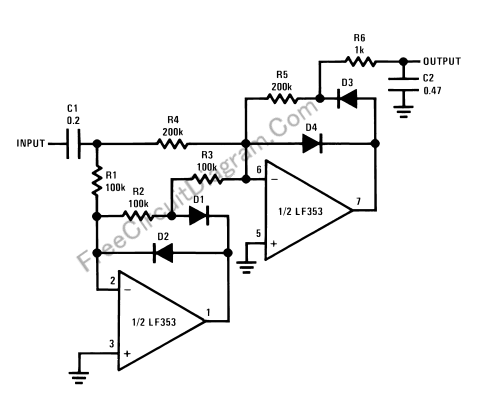# Precision Full-Wave Peak Detector: Detect The Envelope of An Audio SignalThis circuit detect the amplitude of audio signal, suitable for audio level detection that demand for high precision. Amplitude detection or signal envelope detection is usually implemented using a peak detector. An ideal amplitude detector give continuous level at any time because the amplitude of a sine signal (every signal can be seen as sine signal combination) is exist and continuous. I you have a fixed amplitude 100Hz signal for example, an amplitude should give a constant level at any phase, not only when the signal is peaking in the time domain. Here is the circuit’s schematic diagram.For practical reason, many amplitude actually detect only the peak phase of the signal to represent its amplitude, and assume that the amplitude is constant until the detector find the next peaking phase. Simple detector might detect only half cycle of the signal, but here the circuit detect both positive and negative peaking phase, so it would be more precise. Note that the output is  unbuffered, so only a stage with high  impedance input could be connected to this circuit’s output. [Circuit’s schematic diagram source: National Semiconductor Application Notes]